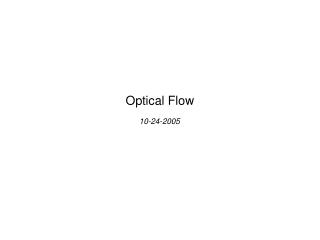DownloadDownload PresentationOptical Flow

# Optical Flow

Download Presentation## Optical Flow

- - - - - - - - - - - - - - - - - - - - - - - - - - - E N D - - - - - - - - - - - - - - - - - - - - - - - - - - -
##### Presentation Transcript

1. Optical Flow 10-24-2005

2. Problem • Problems in motion estimation • Noise, • color (intensity) smoothness, • lighting (shadowing effects), • occlusion, • abrupt movements, etc • Approaches: • Block matching, • Generalized block matching, • Optical flow (block-based, Horn-Schunck etc) • Bayesian, etc. • Applications • Video coding and compression, • Segmentation • Object reconstruction (structure-from-motion) • Detection and tracking, etc.

3. = ì x X í = y Y î Motion description • 2D motion: • p = [x(t),y(t)]p’= [x(t+ t0), y(t+t0)] • d(t) = [x(t+ t0)-x(t),y(t+t0)-y(t)] • 3D motion: • Α= [ Χ1, Υ1, Ζ1 ]ΤΒ = [ Χ2, Υ2, Ζ2 ]Τ • = R+T • Basic projection models: • Orthographic • Perspective

4. Optical Flow • Basic assumptions: • Image is smooth locally • Pixel intensity does not change over time (no lighting changes) • Normal flow: • Second order differential equation:

5. and Block-based Optical Flow Estimation • Optical flow estimation within a block (smoothness assumption): all pixels of the block have the same motion • Error: • Motion equation:

6. Gauss-Seidel Horn-Schunck • We want an optical flow field that satisfies the Optical Flow Equation with the minimum variance between the vectors (smoothness)

7. Derivative Estimation with Finite differences

8. Example 1

9. Example 2

10. Example 3: frame reconstruction

11. Application Examples